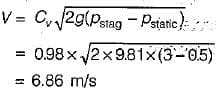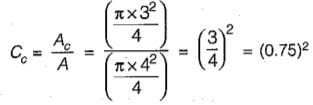Test: Energy Equation & Its Applications - 1

# Test: Energy Equation & Its Applications - 1 - Civil Engineering (CE)

Test Description

## 10 Questions MCQ Test GATE Civil Engineering (CE) 2024 Mock Test Series - Test: Energy Equation & Its Applications - 1

Test: Energy Equation & Its Applications - 1 for Civil Engineering (CE) 2023 is part of GATE Civil Engineering (CE) 2024 Mock Test Series preparation. The Test: Energy Equation & Its Applications - 1 questions and answers have been prepared according to the Civil Engineering (CE) exam syllabus.The Test: Energy Equation & Its Applications - 1 MCQs are made for Civil Engineering (CE) 2023 Exam. Find important definitions, questions, notes, meanings, examples, exercises, MCQs and online tests for Test: Energy Equation & Its Applications - 1 below.
Solutions of Test: Energy Equation & Its Applications - 1 questions in English are available as part of our GATE Civil Engineering (CE) 2024 Mock Test Series for Civil Engineering (CE) & Test: Energy Equation & Its Applications - 1 solutions in Hindi for GATE Civil Engineering (CE) 2024 Mock Test Series course. Download more important topics, notes, lectures and mock test series for Civil Engineering (CE) Exam by signing up for free. Attempt Test: Energy Equation & Its Applications - 1 | 10 questions in 30 minutes | Mock test for Civil Engineering (CE) preparation | Free important questions MCQ to study GATE Civil Engineering (CE) 2024 Mock Test Series for Civil Engineering (CE) Exam | Download free PDF with solutions
 1 Crore+ students have signed up on EduRev. Have you?
Test: Energy Equation & Its Applications - 1 - Question 1

### A Pitot-static tube, with a coefficient of velocity 0.98 is used to measure the velocity of water in a pipe. The stagnation pressure recorded is 3 m and the static pressure is 0.5 m. What is the velocity of flow?

Detailed Solution for Test: Energy Equation & Its Applications - 1 - Question 1Test: Energy Equation & Its Applications - 1 - Question 2

### The integral momentum equation requires the following assumption in the flow

Test: Energy Equation & Its Applications - 1 - Question 3

### A flow through an expanding tube at a constant rate is called

Detailed Solution for Test: Energy Equation & Its Applications - 1 - Question 3

Flow of liquid through a long pipe of constant diameter at a constant rate is steady uniform flow; flow of liquid through a long pipe line of constant diameter, at either increasing or decreasing rate is unsteady-uniform flow; flow of liquid through a tapering pipe at a constant rate is steady-nonuniform flow and flow through a tapering pipe at either increasing or decreasing rate is unsteady- non-uniform flow.

Test: Energy Equation & Its Applications - 1 - Question 4

To avoid the tendency of separation of flow at the throat in a Venturimeter, the ratio of the diameter at the throat to the diameter of the pipe should be

Detailed Solution for Test: Energy Equation & Its Applications - 1 - Question 4

The ratio of throat to inlet diameters d2/d1 may range between 0.75 and 0.25, but the most commonly used ratio is 0.50.
A smaller ratio gives a higher difference in the piezometric heads (between the inlet and the throat) which can be measured more accuratedly by a differential manometer. At the same time, a smaller throat (that is a lower d2/d1 ratio) will mean higher throat velocities which may cause pressures low enough to liberate dissolved gases thereby creating conditions for cavitation to set in.

Test: Energy Equation & Its Applications - 1 - Question 5

Venturimeter is used to measure

Detailed Solution for Test: Energy Equation & Its Applications - 1 - Question 5

A venturi meter is a device which is used for measuring the rate of flow of fluid through a pipe. The basic principle on which a venturi meter works in that by reducing the cross-sectional area of the flow passage, a pressure difference is created and the measurement of the pressure difference enables the determination of the discharge through the pipe.

Test: Energy Equation & Its Applications - 1 - Question 6

A pipe of length more than double the diameter of the orifice fitted internally or externally to the orifice is called

Test: Energy Equation & Its Applications - 1 - Question 7

A point in a compressible flow where the velocity of fluid is zero, is called

Detailed Solution for Test: Energy Equation & Its Applications - 1 - Question 7

At vena-contracta velocity of fluid is maximum at stagnation point velocity is zero and all kinetic energy is converted to pressure energy.

Test: Energy Equation & Its Applications - 1 - Question 8

The equation P/w + V2/2g + Z = constant is based on the following assumptions regarding the flow of fluid:

Detailed Solution for Test: Energy Equation & Its Applications - 1 - Question 8

The equation is Bernoulli’s equation. It is based on following assumptions:
(ii) fluid is incompressible
(iii) fluid is. non-viscous since viscous force has been neglected; and
(iv) it is applicable to points along a streamline

Test: Energy Equation & Its Applications - 1 - Question 9

A fluid jet discharging from a 4 cm diameter orifice has a diameter 3 cm at its vena contracta. If the coefficient of velocity is 0.98, the coefficient of discharge for the orifice will be

Detailed Solution for Test: Energy Equation & Its Applications - 1 - Question 9

For an orifice the coefficient of discharge Cd, coefficient of velocity Cv and coefficient of contraction Cc is related as,
Cd = Cc x CvCd = (0.75)2 x 0.98

Test: Energy Equation & Its Applications - 1 - Question 10

The coefficient of velocity for an orifice is given by (using usual notation)

## GATE Civil Engineering (CE) 2024 Mock Test Series

28 docs|296 tests
Information about Test: Energy Equation & Its Applications - 1 Page
In this test you can find the Exam questions for Test: Energy Equation & Its Applications - 1 solved & explained in the simplest way possible. Besides giving Questions and answers for Test: Energy Equation & Its Applications - 1, EduRev gives you an ample number of Online tests for practice

## GATE Civil Engineering (CE) 2024 Mock Test Series

28 docs|296 tests